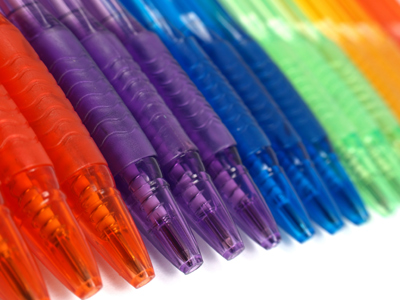No need for a pen and paper I hope - see if you can work out the answers in your head.

# Fractions 2 (Medium)

Welcome to this, the second of our medium level quizzes on fractions. In it we shall recap what we have learnt about denominators, numerators, mixed and improper fractions. These are all important parts of Eleven Plus maths, so you need to understand them before you take your exams.

Fractions, as you know, are a way of representing parts of numbers. They do exactly the same job as decimal numbers, but in a very different way! For example, one-half as a decimal is 0.5, but as a fraction it’s written 12. That simply means the top number (1) ÷ the bottom number (2).

Just to make sure you know, the top number in a fraction is the NUMERATOR and the bottom number is the DENOMINATOR. Denominators (the 2 in 12) tell us how many pieces to cut a whole into, while numerators tell us how many of these pieces we get.

I’m sure by now that you are a whizz with fractions. So, have a go at this quiz and see if you can get 1010 of the questions right!

1.
How many nineteenths are there in 3?
60
57
54
22
There are 19 nineteenths in 1, so there are 19 × 3 = 57 nineteenths in 3. In general, the number in the denominator tells you how many fractions of that type are needed to make 1. For example, you need three thirds, 1⁄3, to make 1, but you need ten tenths, 1⁄10, to make 1 and so on. REMEMBER this stuff, and you won't get confused with fractions
2.
What is 184 as a mixed fraction?
4
4 14
4 12
4 34
To convert an improper fraction to a mixed fraction, follow these steps: 1. Divide the numerator by the denominator. 2. Note the whole number remainder. 3. Write the number from step 1 as the whole number in front of the fractional part AND write the fractional part with the remainder in the numerator and keep the original denominator. STEP 1: 18 ÷ 4 = 4. STEP 2: Remainder 2. STEP 3: 4 24. 4 24 can be simplified by dividing the denominator and numerator by the same number, in this case 2, to give 4 12
3.
What is 7 1421 as an improper fraction?
243
15021
537
233
To convert a mixed fraction to an improper fraction, follow these steps: 1. Multiply the whole number part by the denominator. 2. Add this result to the numerator. 3. Write the fraction with step 2 in the numerator and keep the original denominator. STEP 1: 7 × 21 = 147. STEP 2: 147 + 14 = 161. STEP 3: 16121. This can then be simplified by dividing denominator and numerator by the same number, in this case 7, to give 233
4.
What is 23 + 46 + 89?
2 23
2 79
3
2 29
First, convert all the fractions to the same numerator: 23 + 46 + 89 = 69 + 69 + 89 = 209. We then convert this into a mixed fraction: 2 29
5.
Which is greater: 17 x 14, or 19 x 13?
19 x 13
17 x 14
They are equal in value
To multiply fractions, times the numerators by each other, and the denominators by each other: 17 x 14 = 128
19 x 13 = 127
6.
What is 97 of 49?
56
63
70
77
Of means multiply in this case: 97 × 49 = (9 × 49) ÷ 7 = 441 ÷ 7 = 63. 97 is greater than 1, so the answer will be more than 49
7.
What is 910 - 25 + 32?
2 115
1 45
2 110
2
910 - 25 is the same as 910 - 410 = 510. 510 can be simplified by dividing denominator and numerator by the same number, in this case 5, to give 12. Then 12 + 32 = 42. Simplify this by dividing denominator and numerator by the same number, in this case 2, to give 20 = 2
8.
What is 45 of 45?
42
40
36
32
Of means multiply in this case: 45 × 45 = (4 × 45) ÷ 5 = 180 ÷ 5 = 36. Do the brackets first.
9.
What is 5213 as a mixed fraction?
4
3 1213
4 113
2
To convert an improper fraction to a mixed fraction, follow these steps: 1. Divide the numerator by the denominator. 2. Note the whole number remainder. 3. Write the number from step 1 as the whole number in front of the fractional part AND write the fractional part with the remainder in the numerator and keep the original denominator. STEP 1: 52 ÷ 13 = 4. STEP 2: Remainder 0. STEP 3: 4. If there is no remainder then the answer will be a whole number
10.
What is 4 78 as an improper fraction?
92
398
194
378
To convert a mixed fraction to an improper fraction, follow these steps: 1. Multiply the whole number part by the denominator. 2. Add this result to the numerator. 3. Write the fraction with step 2 in the numerator and keep the original denominator. STEP 1: 4 × 8 = 32. STEP 2: 32 + 7 = 39. STEP 3: 398
Author:  Frank Evans